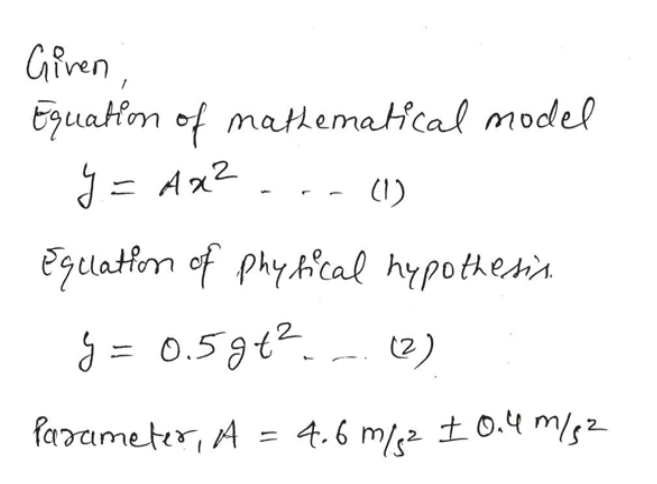suppose you collected a data set in which you measured fall-times for different fall-heights, you plotted the data and fit the mathemathical model, y=Ax^2, to match the physical hypothesis, y=0.5*g*t^2. From the best fit curve you are told that the value of your fit-parameter, A, is for 4.6 m/s^2 +/- 0.4 m/s^2. Determine the value of g +/- delta g for this fit parameter value.

Question

suppose you collected a data set in which you measured fall-times for different fall-heights, you plotted the data and fit the mathemathical model, y=Ax^2, to match the physical hypothesis, y=0.5*g*t^2. From the best fit curve you are told that the value of your fit-parameter, A, is for 4.6 m/s^2 +/- 0.4 m/s^2. Determine the value of g +/- delta g for this fit parameter value.

Step 1help_outlineImage TranscriptioncloseGfven Eguatom of matematical model J=Ax2 Egulatiem of phycal hypothesi 2) farameter, A 4.6 ms2 0.4 m/s fullscreen

Want to see the full answer?

See Solution

Want to see this answer and more?

Our solutions are written by experts, many with advanced degrees, and available 24/7

See Solution
Tagged in

Physics